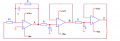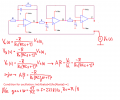# Opamp oscillator

#### xxxyyyba

Joined Aug 7, 2012
289
Hello.In oscillator circuit ideal opamps are used, it is known R=10K, C=10nF.
a) Calculate frequency of oscillations,
b) If Vcc=Vee=15V, calculate amplitude of voltages in points A, B and C.
a)
Here is my solution. I cut output loop, applied test generator and found loop gain on this way:I cut loop on output, because they did same in this type of exercises. Is it general rule that to find loop gain Abeta, we cut loop on output?
How to solve task b) ?

#### Jony130

Joined Feb 17, 2009
5,475
Is it general rule that to find loop gain Abeta, we cut loop on output?
Yes.

As for b). The linear theory of oscillation do not give as any info about the amplitude and your circuit do not have any circuit for amplitude stabilization. So what you can do is simply assume that Va is 30Vpeak-peak and solve for Vb and Vc.

•xxxyyyba